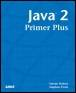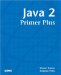# Review QuestionsJava™ 2 Primer PlusBy Steven Haines, Steve Potts Table of Contents Chapter 3.  Operators

 1: What is modulus operator, and how is it used? 2: What is the difference between the following operators: && and &? 3: What is a truth table, and what does it tell you? 4: What is the result of 21 & 3? 5: What is the result of 21 | 3? 6: What is the result of 21 ^ 3? 7: What is the difference between the following operators: >> and >>>? 8: What is the result of 5 * 5 + 5? 9: What is the result of 5 + 5 * 5? 10: What is the result of the following code fragment? ` int a = 5; a += 6 * ++a / 2 6 * 9 + 2 `

 TopJava 2 Primer Plus
ISBN: 0672324156
EAN: 2147483647
Year: 2001
Pages: 332

Similar book on Amazon